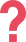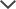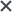Note: the PARCC Practice Pack is NOT included in our Premium Membership.

The Mathematics test presents students with multi-step problems that require mathematical reasoning and understanding to solve. The test also asks students to apply mathematical concepts and equations to solve real-world problems. To pass the test, students must demonstrate that they have mastered the key knowledge and skills for their grade level as identified by the Common Core State Standards (CCSS).

## PARCC Scoring Rubric

The scoring rubric describes the level of achievement a response demonstrates for each score point. The rubric provides specific insight per item and measures students' mathematical understanding by assessing the solution process, modeling, and reasoning. The correct application and comprehension of various aspects of mathematical principles and concepts are also tested.

Aside from the explanation, the scoring rubric also provides a range of score points for each item. Scorers are provided with examples that serve as a reference and which ensure they interpret rubrics consistently and correctly. Learn more about the PARCC scores.

## PARCC Question Types

Students can expect to encounter three types of questions on the PARCC test:

• Type I – Questions assessing concepts, skills, and procedures. These questions balance conceptual understanding, fluency, and application, and involve any or all mathematical applications. Type I questions vary in format and are machine scorable.
• Type II – Questions assessing the expression of mathematical reasoning. Students must present written arguments for and justifications of their solutions. They must demonstrate critical reasoning and precision in mathematical statements. Type II questions may include a mix of machine-scored and hand-scored responses.
• Type III – Questions assessing modeling and applications of mathematical concepts in a real-world context or scenario. These questions may be used to assess any mathematical concept taught in the classroom. Type III questions may include a mix of machine-scored and hand-scored responses.

• Third Grade – 43 questions in total. Topics covered: products and quotients; multiplication and division; two-step problems; fraction equivalence; fractions as numbers; time; volumes and masses; geometric measurement; multi-digit arithmetic; scaled graphs; measurement data; understanding shapes; perimeter and area; properties of operations; concrete referents and diagrams; distinguishing the correct explanation or reasoning from that which is flawed; and modeling.
• Type I: 36 questions in total, worth 40 points overall. 32 questions are worth 1 point each and 4 questions are worth 2 points each.
• Type II: 4 questions in total, worth 14 points overall. 2 questions are worth 3 points each and 2 questions are worth 4 points each.
• Type III: 3 questions in total, worth 12 points overall. 2 questions are worth 3 points each and 1 question is worth 6 points.
• Fourth Grade– 40 questions in total. Topics covered: fractions and decimals; building fractions; multiplying fractions; solving with multiplication; multi-step problems; place value; addition and subtraction; operations and factors; measurement and conversion; representing and interpreting data; geometric measurement; lines, angles, and shapes; generating and analyzing patterns; properties of operations; concrete referents and diagrams; distinguishing the correct explanation or reasoning from that which is flawed; and modeling.
• Type I: 33 questions in total, worth 40 points overall. 26 questions are worth 1 point each and 7 questions are worth 2 points each.
• Type II: 4 questions in total, worth 14 points overall. 2 questions are worth 3 points each and 2 questions are worth 4 points each.
• Type III: 3 questions in total, worth 12 points overall. 2 questions are worth 3 points each and 1 question is worth 6 points.
• Fifth Grade – 40 questions in total. Topics covered: addition and subtraction operations with decimals; adding and subtracting in context with fractions; fractions with unlike denominators; multiplication and division operations with decimals; multiplying with whole number; quotients and dividends; and multiplying and dividing with fractions.

The rest of the questions cover topics such as: interpreting fractions; recognizing volume; finding volume; reading, writing, and comparing decimals; place value; multiplication scaling; writing and interpreting numerical expressions; graphing on the coordinate plane; two-dimensional figures; conversions; data displays; properties of operations; place value; concrete referents and diagrams; distinguishing the correct explanation or reasoning from that which is flawed; and modeling.
• Type I: 33 questions in total, worth 40 points overall. 26 questions are worth 1 point each and 7 questions are worth 2 points each.
• Type II: 4 questions in total, worth 14 points overall. 2 questions are worth 3 points each and 2 questions are worth 4 points each.
• Type III: 3 questions in total, worth 21 points overall. 2 questions are worth 3 points each and 1 question is worth 6 points.
• Sixth Grade – 38 questions in total. Topics covered: multiplying and dividing with fractions; ratios; rational numbers; expressions and inequalities; factors and multiples; geometry; statistics and probability; operations with multi-digit numbers; properties of operations; concrete referents and diagrams; distinguishing the correct explanation or reasoning from that which is flawed; and modeling.
• Type I: 31 questions in total, worth 40 points overall. 24 questions are worth 1 point each, 6 questions are worth 2 points each, and 1 question is worth 4 points.
• Type II: 4 questions in total, worth 14 points overall. 2 questions are worth 3 points each and 2 questions are worth 4 points each.
• Type III: 3 questions in total, worth 12 points overall. 2 questions are worth 3 points each and 1 question is worth 6 points.
• Seventh Grade – 38 questions in total. Topics covered: proportional relationships; operations with fractions; expressions, equations, and inequalities; representing geometric figures; drawings and measurement; random sampling and comparative inferences; chance processes and probability models; properties of operations; concrete referents and diagrams; distinguishing the correct explanation or reasoning from that which is flawed; and modeling.
• Type I: 31 questions in total, worth 40 points overall. 24 questions are worth 1 point each, 6 questions are worth 2 points each, and 1 question is worth 4 points.
• Type II: 4 questions in total, worth 14 points overall. 2 questions are worth 3 points each and 2 questions are worth 4 points each.
• Type III: 3 questions in total, worth 12 points overall. 2 questions are worth 3 points each and 1 question is worth 6 points.
• Eighth Grade – 36 questions in total. Topics covered: expressions and equations; scientific notation; proportional relationships and linear equations; solving linear equations; simultaneous linear equations; functions; congruence and similarity; Pythagorean theorem; rational numbers; modeling with functions; volume; bivariate data; graphs and equations; reasoning; geometric reasoning; and modeling.
• Type I: 29 questions in total, worth 40 points overall. 22 questions are worth 1 point each, 5 questions are worth 2 points each, and 2 questions are worth 4 points each.
• Type II: 4 questions in total, worth 14 points overall. 2 questions are worth 3 points each and 2 questions are worth 4 points each.
• Type III: 3 questions in total, worth 12 points overall. 2 questions are worth 3 points each and 1 question is worth 6 points.

### PARCC Algebra I

The PARCC Algebra I test contains 42 questions in total. It assesses the following topics: expressions; interpreting functions; rate of change; solving algebraically; solving graphically; number systems; equivalent expressions and functions; interpreting graphs of functions; function transformations; multiple representations of functions; summarizing, representing, and interpreting data; reasoning; and modeling.

• Type I: 34 questions in total, worth 49 points overall. 23 questions are worth 1 point each, 9 questions are worth 2 points each, and 2 questions are worth 4 points each.
• Type II: 4 questions in total, worth 14 points overall. 2 questions are worth 3 points each and 2 questions are worth 4 points each.
• Type III: 4 questions in total, worth 18 points overall. 2 questions are worth 3 points each and 2 question is worth 6 points.

### PARCC Algebra II

The PARCC Algebra II test contains 41 questions in total. It assesses the following topics: equivalent expressions; interpreting functions; rate of change; building functions; statistics and probability; interpreting functions; equivalent expressions; function transformations; trigonometry; solving equations and systems; data – univariate and bivariate; inference; probability; reasoning; and modeling.

• Type I: 33 questions in total, worth 49 points overall. 21 questions are worth 1 point each, 10 questions are worth 2 points each, and 2 questions are worth 4 points each.
• Type II: 4 questions in total, worth 14 points overall. 2 questions are worth 3 points each and 2 questions are worth 4 points each.
• Type III: 4 questions in total, worth 18 points overall. 2 questions are worth 3 points each and 2 question is worth 6 points.

### PARCC Geometry

The PARCC Geometry test contains 43 questions in total. It assesses the following topics: congruence transformations; similarity; similarity in trigonometry; modeling and applying; transformations; geometric constructions; applying geometric properties and theorems; geometric formulas; reasoning; and modeling.

• Type I: 35 questions in total, worth 49 points overall. 25 questions are worth 1 point each, 8 questions are worth 2 points each, and 2 questions are worth 4 points each.
• Type II: 4 questions in total, worth 14 points overall. 2 questions are worth 3 points each and 2 questions are worth 4 points each.
• Type III: 4 questions in total, worth 18 points overall. 2 questions are worth 3 points each and 2 question is worth 6 points.

### PARCC Integrated Math I

The PARCC Integrated Math I test contains 42 questions in total. It assesses the following topics: expressions and equations; rate of change; interpreting functions; solving graphically; congruence transformations; summarizing, representing, and interpreting data; transformations; solving systems; contextual problem functions; reasoning; and modeling.

• Type I: 34 questions in total, worth 49 points overall. 23 questions are worth 1 point each, 9 questions are worth 2 points each, and 2 questions are worth 4 points each.
• Type II: 4 questions in total, worth 14 points overall. 2 questions are worth 3 points each and 2 questions are worth 4 points each.
• Type III: 4 questions in total, worth 18 points overall. 2 questions are worth 3 points each and 2 question is worth 6 points.

### PARCC Integrated Math II

The PARCC Integrated Math II test contains 42 questions in total. It assesses the following topics: quadratics and exponential expressions; quadratic equations; graphing exponential and quadratic functions; rate of change; polynomial, rational, and radical expressions; similarity; similarity in trigonometry; probability; statistics; geometric formulas; graphs; multiple representations of functions; number systems; reasoning; and modeling.

• Type I: 34 questions in total, worth 49 points overall. 23 questions are worth 1 point each, 9 questions are worth 2 points each, and 2 questions are worth 4 points each.
• Type II: 4 questions in total, worth 14 points overall. 2 questions are worth 3 points each and 2 questions are worth 4 points each.
• Type III: 4 questions in total, worth 18 points overall. 2 questions are worth 3 points each and 2 question is worth 6 points.

### PARCC Integrated Math III

The PARCC Integrated Math III test contains 36 questions in total. It assesses the following topics: equivalent expressions; interpreting functions; rate of change; solving equations; modeling with geometry; statistics and probability; interpreting functions; expressions and equations; function transformations; trigonometry; data – univariate and bivariate; inference; properties and theorems; geometric constructions; reasoning; and modeling.

• Type I: 29 questions in total, worth 40 points overall. 22 questions are worth 1 point each, 5 questions are worth 2 points each, and 2 questions are worth 4 points each.
• Type II: 4 questions in total, worth 14 points overall. 2 questions are worth 3 points each and 2 questions are worth 4 points each.
• Type III: 3 questions in total, worth 12 points overall. 2 questions are worth 3 points each and 1 question is worth 6 points.

## How to Prepare for the PARCC Math

Here are four tips to help you ace the PARRC Mathematics assessment – MATH:

• Master the concepts. Memorizing math isn't useful when it comes to applying concepts to different problems. Math is a tool that must be mastered to be properly wielded, so encourage your child to understand the rationale behind the math. Comprehension of mathematical concepts will improve your child's learning process and problem-solving skills.
• Apply math in everyday life. PARCC questions ask students to apply mathematical concepts and reasoning to solve real-world problems, so the best way to practice is to try and apply math to everyday problems as well.
• Turn weaknesses into strengths. Find the mathematical concepts, techniques, or applications that challenge your child and tackle them head on. Mastering a difficult concept will increase your child's confidence as well as improve his or her grade in the class.
• Hard work pays off. Solve problems in a step-by-step and tidy manner to avoid any mistakes, illustrate the thought process and problem-solving strategy used, and understand the mathematical concepts better. When the steps are clearly written, it is easy to spot recurring mistakes and to learn from them.

## PARCC Practice for Math - Online

The PARCC Math test reviews the mathematical concepts students have learned throughout the year, and it plays a significant role in influencing your child's future. That is why it is so vital to spend time preparing for it. TestPrep-Online's PARCC preparation packs contain PARCC practice tests, study guides, and detailed answer explanations, all of which will help prepare your child for the PARCC.

The PARCC and other trademarks are the property of their respective trademark holders. None of the trademark holders are affiliated with TestPrep-Online or this website.

Need HelpNeed Help